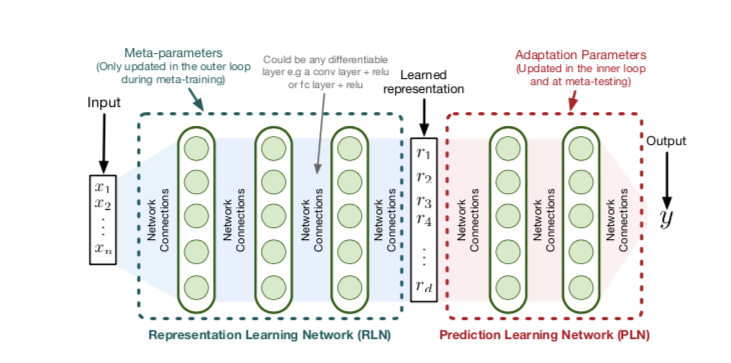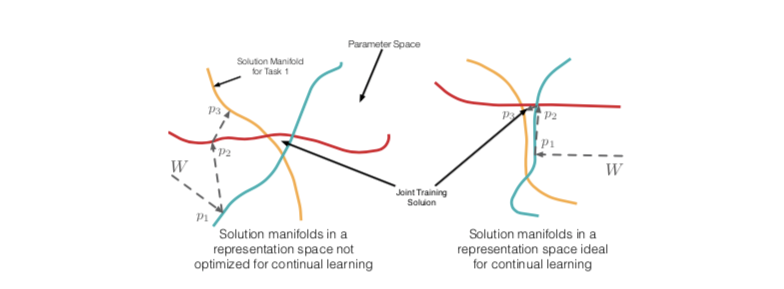# Meta-Learning Representations for Continual Learning

## Problem Formulation

Continual Learning Prediction (CLP)问题由无休止的样本流组成

## MethodOML的目标函数为 ：

$U(Wt, \theta, S_k^j) =(W{t+k},\theta)$ 表示一个更新 $W_{t+k}$ 是k步SGD后的权重向量

U 中第 $j$ 步更新 在样本 $(X{t+j}^i, Y{t+j}^i)$ 使用参数 $W{t+j-1}, \theta$ , 得到 $(W{t+j},\theta)$

MAML-Rep和OML目标可以分别实现为算法1和算法2，两者之间的主要区别以蓝色突出显示:OML 目标的目的是学习适合在线持续学习的表示。 为了说明什么将构成持续学习的有效表示，假设我们有三个输入集群，它们具有显着不同的 $p(Y |x)$，对应于 $p1$、$p_2$ 和 $p_3$。 对于固定的二维表示 $\phi{\theta} : X \to R^2$，我们可以考虑由线性模型给出的解 $W\in R^2$ 的线性模型，该模型为每个 $p_i$ 提供等效准确的解。